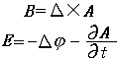1)  Electromagnetic potentialequation2)  electromagnetic equations1.
And according to the varied work states,the corresponding electromagnetic equations were listed.

3)  Electromotive force equation1.
The electromotive force equations of salient pole synchronous motor are deduced by two-reaction theory and by coupling circuit concept.

2.
The electromotive force equation in static state of transformer、DC motor、asynchronous motor、synchronous motor without damping windings were deduced by theory of composite magnetic field in all kinds of teaching material about electric machinery,but in dynamic state the coupling circuit method from electric theory was adopted.

4)  electromagnetic field equations5)  electromagnetic field equatio1.
And the way of using the electromagnetic field equation of transformer to overcome the defect in the conventional approach with second harmonic of traction transformer is theoretically discussed.

6)  Electromagnetic Wave Equation1.
Using the electromagnetic wave equation in gravitational field,we give a general method of calculating the deflection of light by gravitational field under the condition of weak field approximation.

 电磁势electromagnetic potentials   描述电磁场的基本物理量。它包括矢势A和标势j两部分，在电磁理论中起着十分重要的作用。   在经典的电磁场理论中，描述电磁场的基本物理量是电t场强度E和磁感应强度B，根据矢量分析，任何无源场可以表为某个矢量场的旋度，因磁场无源即Δ·B＝0，故可引入矢势A和标势j，定义为说明：补充资料仅用于学习参考，请勿用于其它任何用途。 参考词条 ©2011 dictall.com